# Infinity facts for kids

Kids Encyclopedia Facts

Infinity is about things which never end. Sometimes, it is also written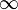$\infty$. Infinity means many different things, depending on when it is used. The word is from a Latin word, which means "without end". Infinity goes on forever, so sometimes space, numbers, and other things are said to be 'infinite', because they never come to a stop.

Infinity is not really an ordinary number, but it is sometimes used as one. Infinity often says how many there is of something, instead of how big something is. For example, there are infinitely many whole numbers (called integers), but there is no integer which is infinitely big. But different kinds of math have different kinds of infinity. So its meaning often changes.

There are two kinds of infinity: potential infinity and actual infinity. Potential infinity is a process that never stops. For example, adding 10 to a number. No matter how many times 10 is added, 10 more can still be added. Actual infinity is a more abstract idea. For example, there are infinitely many numbers as it is impossible to write them all down.

## Infinity in Mathematics

Mathematicians have different sizes of infinity and three different kinds of infinity.

### Counting infinity

The number of things, beginning with 0, 1, 2, 3, ..., to include infinite cardinal numbers. There are many different cardinal numbers. Infinity can be defined in one of two ways: Infinity is a number so big that a part of it can be of the same size; Infinity is larger than all of the natural numbers. There is a smallest infinite number, countable infinity. It is the counting number for all of the whole numbers. It is also the counting number of the rational numbers. The mathematical notation is the Hebrew letter aleph with a subscrpt zero;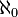$\aleph_0$. It is spoken "aleph null". It was a surprise to learn that there are larger infinite numbers. The number of real numbers, that is all numbers with decimals, is larger than the number of rational numbers, the number of fractions. This shows that there are real numbers which are not fractions. The smallest infinite number greater than$\aleph_0$ is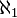$\aleph_1$. The number of mathematical functions is the next infinite cardinal number,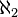$\aleph_2$. And these numbers go on without end.

### Ordering infinity

A different type of infinity are the ordinal numbers, beginning "first, second, third, ...". The order "first, second, third, ..." and so on to infinity is different from the order ending "..., third, second, first". The difference is important for mathematical induction. The simple first, second, third, ... has the mathematical name: the Greek letter omega with subscript zero: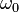$\omega_0$. (Or simply omega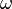$\omega$.) The infinite series ending "... third, second, first" is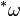$^*\omega$.

### The real line and complex plane

The third type of infinity has the symbol$\infty$. This is treated as addition to the real numbers or the complex numbers. It is the result of division by zero, or to indicate that a series is increasing (or decreasing) without bound. The series 1, 2, 3, ... increases without upper bound. This is written: the limit is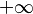$+\infty$. In calculus, the integral over all real numbers is written: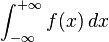$\int_{-\infty}^{+\infty} f(x)\,dx$

## The arithmetic of infinity

Each kind of infinity has different rules.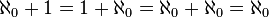$\aleph_0 + 1 = 1 + \aleph_0 = \aleph_0 + \aleph_0 = \aleph_0$ Addition with "alephs" is commutative.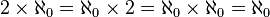$2 \times \aleph_0 = \aleph_0 \times 2 = \aleph_0 \times \aleph_0 = \aleph_0$ Multiplication with "alephs" is commutative.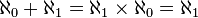$\aleph_0 + \aleph_1 = \aleph_1 \times \aleph_0 = \aleph_1$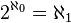$2 ^ {\aleph_0} = \aleph_1$.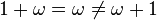$1 + \omega = \omega \neq \omega +1$. Addition with "omegas" is not commutative.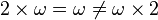$2 \times \omega = \omega \neq \omega \times 2$. Multiplication with "omegas" is not commutative.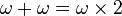$\omega + \omega = \omega \times 2$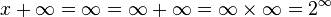$x + \infty = \infty = \infty + \infty = \infty \times \infty = 2 ^ \infty$

### Subtraction, division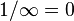$1 / \infty = 0$ Otherwise, division by infinity is not meaningful. Subtraction with infinity is not meaningful.

## Images for kidsInfinity Facts for Kids. Kiddle Encyclopedia.